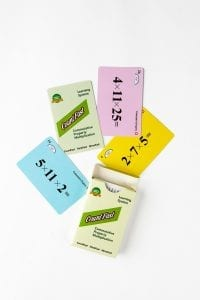# Commutative Property Multiplication Home Activites

####OVERVIEW & PURPOSE

Week 7 of the 2nd Grade CountFast program reviews using the Commutative Property of Addition to quickly solve equations with more than two numbers. This module includes equations to solve each day, with an emphasis on improving fluency and speed with basic facts. Spend 15 minutes each day on one of the activities listed in this module.  Card decks should go home with students each day for additional practice with a parent at home. Each week, a new deck is introduced and the previous deck is for the student to keep at home for continued practice.

#### EDUCATION STANDARDS

1.  NCTM Standard: develop fluency with basic number combinations for addition and subtraction
2.  NCTM Standard: understand the effects of adding and subtracting whole numbers
3.  CCSS.MATH.CONTENT.1.OA.C.6  Add and subtract within 20, demonstrating fluency for addition and subtraction within 10.

#### OBJECTIVES

Develop fluency in using the Commutative Property concept to quickly mentally calculate single-digit addition and subtraction equations.

DAYS 1 through 3:

Each day, we will practice quickly adding up problems that have three addends by looking for easy ways to pair the numbers.  Use the yellow cards from the deck.  These have addition equations on them.  Hold up one equation at a time for your child to read and answer. (If you are holding the card, 1 + 5 + 9 =, your child might say “One plus five equals 6, and nine more makes 15.”)  Remind your child of the Commutative Property of Addition, and how this allows them to add up the numbers in any order to get the same sum.  Look especially for way to make a group of 10 to make calculation easier.  The same card from above can be solved more quickly by saying, “One and nine make 10, and ten plus five makes 15.”  Practice together using the yellow cards each day.  For a challenge, you can use the “Time Record Card” in the deck to track the time it takes your child to correctly complete all of the yellow cards.  Encourage your child to complete the cards a little faster each day.

DAYS 4 and 5:

Use the BLUE cards from the deck.  These have subtraction equations on them.  Hold up one equation at a time for your child to read and answer. For cards with three digits in the equation, explain that the most important part of the problem is remembering that the beginning (1st) number is what you have to start with.  The other digits can be subtracted from that number in any order.  For example: 20 – 1 – 9 = 10.  This can be solved in left-to-right order by subtracting 1 from 20 to get 19, and then subtracting 9 from the remaining 19 to get a final difference of 10.  However, another strategy is to first add the 1 and 9 together to make 10, and then subtracting that total of 10 from the original number of 20.  Additionally, this can be solved by first subtracting the 9 from the 20 to get 11, and then subtracting the 1 to get a final difference of 10. Remind your child that solutions can be made faster by looking for ways to group numbers to make 10.  Practice together using the BLUE cards.  For a challenge, you can use the “Time Record Card” in the deck to track the time it takes your child to correctly complete all of the blue cards.  Encourage your child to complete the cards a little faster each day.

Stay SharpFree flash card decks to keep kids entertained and sharp!

To help you keep your kids math sharp during this crisis, we're giving you 3 free CountFast flash card decks for just \$6 in shipping.  Just have them take the quick quiz for their grade at the link below and you'll get a discount code to use on any three decks.